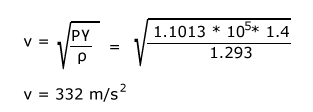# Velocity of Sound in Gas (Newton’s formula)

According to Newton, when sound waves propagate in air, compression and rarefaction are formed. He assumed that the process is very slow and the heat produced during compression is given to surrounding and heat loss during compression is gained from surrounding. So the temperature remains constant and sound waves propagate through an isothermal process.

## Derivation of Newton’s Formula

According to gas law (Boyle’s law),

```PV = constant
where, P = pressure
V = volume of air
```

Differentiating above equation, we get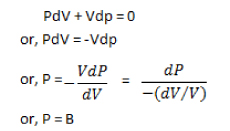where, B is the bulk modulus of the air.

If B is the bulk modulus of the air, v is velocity and ρ is the density, then, velocity is given by: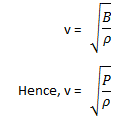This is the required expression for velocity of sound in air.

### Calculation of Velocity of air

Now, the velocity of sound in air using Newton’s formula at NTP (Normal Temperature and Pressure) is given by:

```Pressure (P)= 1.1013 * 105 N/m2
Density of air (ρ) = 1.293 kg/m3
```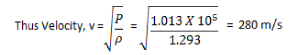Experimentally it has been found that the velocity of sound in air is 332 m/s. We can conclude that, there is some error in Newton’s assumption and it needs correction.

## Laplace Correction of Newton’s Formula

Laplace provided a correction for Newton’s formula. He assumed that, the process of compression and rarefaction occur very fast and no exchange of heat takes place. Thus, the temperature doesn’t remain constant and the propagation of sound in gas is an adiabatic process.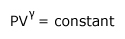```where,
γ = Cp/Cv
Cp = Specific heat for constant pressure
Cv = Specific heat for constant volume
```

Differentiating the equation, we get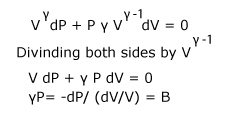If B is the bulk modulus of the air, v is velocity, γ is the adiabatic index and ρ is the density, then, velocity of sound is gived by is given by: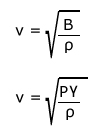### Calculation of Velocity of Sound with Laplace Correction

Now, the velocity of sound in air using Laplace correction of Newton’s formula at NTP (Normal Temperature and Pressure) is given by:

```Pressure (P)= 1.1013 * 105 N/m2
Density of air (ρ) = 1.293 kg/m3